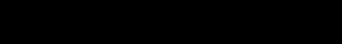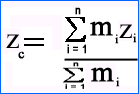# Centre Of Mass

Definition
The centre of mass of a body or a system of particles is defined as
“A single point at which the whole mass of the body or system is
imagined to be concentrated and all the applied forces acts at that point.”

Explanation
In translational motion each point on a body undergoes the same displacement as any other point as time passes, so that motion of one particle represents the motion of the whole body. But when a body rotates or vibrates, there is one point on the body, called “centre of mass”, that moves in the same way that a single particle would move under the influence of the same external forces.
Centre of mass of a body is that point that moves when forces are applied on the body. The motion of a body can be described as the motion of its centre of mass.
The centre of mass , has translational motion under the influence of forces. If a single force acts on a body and the line of action of the force passes through the centre of mass, the body will have linear acceleration but possess no angular acceleration.

Centre of mass and centre of gravity
The centre of mass often confused with the centre of gravity. The two terms are so similar in many respects that one can use the two interchangeably. The centre of gravity of an extended object coincides with its centre of mass if the object is in a completely uniform gravitational field. If the body is not located in a uniform gravitational field, its centre of mass and centre of gravity will be at two different locations.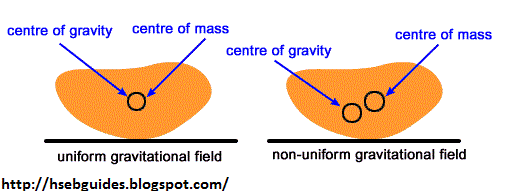Centre of mass of different objects
Centre of mass of a body depends on its geometrical shape. If a body is symmetrical and of uniform composition, the centre of mass will be located at its geometrical centre.
For example:

• Centre of mass of a square is at the point of intersection of its diagonals.
• Centre of mass of a sphere is at its centre.
• Centre of mass of a rigid bar is at the middle point.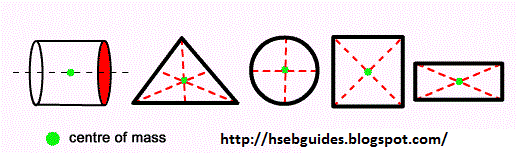Determination of centre of mass
Consider a system of “n” particles of masses: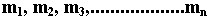of body whose position vectors are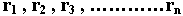position vectors respectively.

The centre of mass of the system is defined as the point having the position vector “r” and is equal to:Where M is the total mass of the system.
If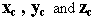are the coordinates of the centre of mass of a system of “n” particles then,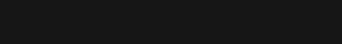Or,Also,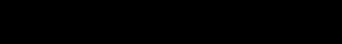Or,Similarly,